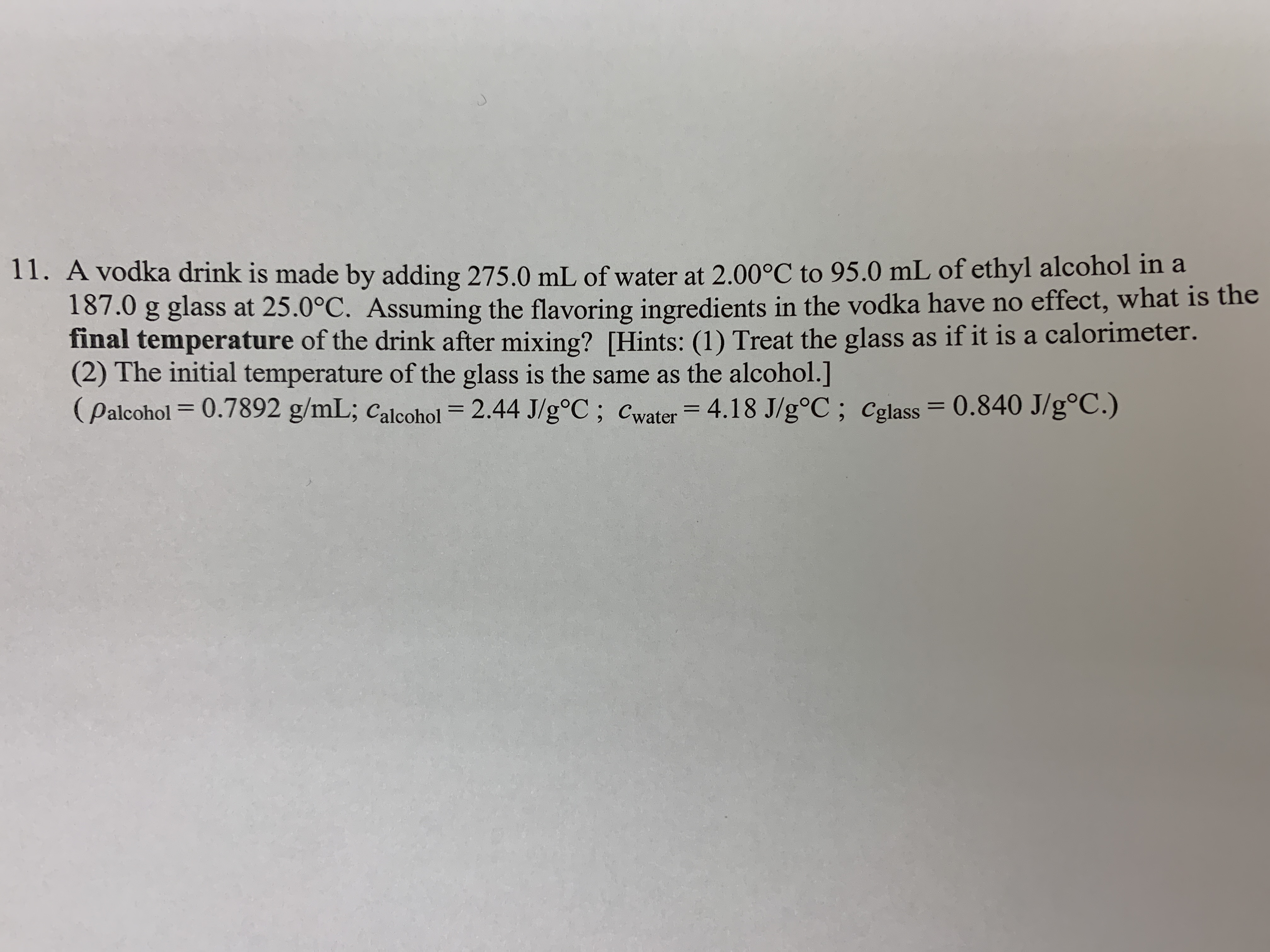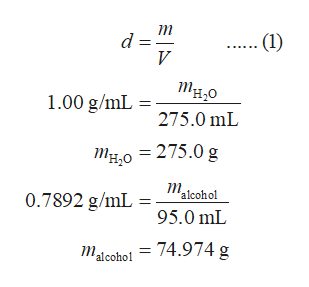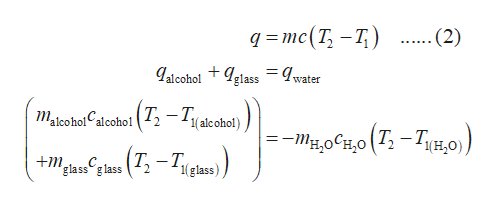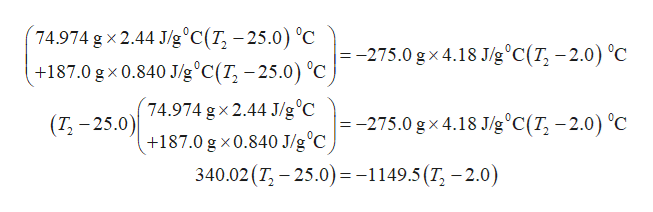# 11. A vodka drink is made by adding 275.0 mL of water at 2.00°C to 95.0 mL of ethyl alcohol in a187.0 g glass at 25.0°C. Assuming the flavoring ingredients in the vodka have no effect, what is thefinal temperature of the drink after mixing? [Hints: (1) Treat the glass as if it is a calorimeter.(2) The initial temperature of the glass is the same as the alcohol.](Palcohol = 0.7892 g/mL; calcohol = 2.44 J/g°C; cwater = 4.18 J/g°C ; cglass = 0.840 J/g°C.)

Question
14 viewshelp_outlineImage Transcriptionclose11. A vodka drink is made by adding 275.0 mL of water at 2.00°C to 95.0 mL of ethyl alcohol in a 187.0 g glass at 25.0°C. Assuming the flavoring ingredients in the vodka have no effect, what is the final temperature of the drink after mixing? [Hints: (1) Treat the glass as if it is a calorimeter. (2) The initial temperature of the glass is the same as the alcohol.] (Palcohol = 0.7892 g/mL; calcohol = 2.44 J/g°C; cwater = 4.18 J/g°C ; cglass = 0.840 J/g°C.) fullscreen
check_circle

Step 1

The mass of water and ethyl alcohol present in the system is calculated using equation (1). In this equation, d represents the density, m represents the mass and V represents the volume of the substance.help_outlineImage Transcriptioncloseт (1) тно 1.00 g/mL = 275.0 mL Mg,0 = 275.0 g m, 0.7892 g/mL =: 'alcohol 95.0 mL = 74.974 g Malcohol fullscreen
Step 2

In the given system, water is at 2.00 0C and ethyl alcohol in glass is at 25.0 0C. Therefore, the total amount of heat lost by ethyl alcohol and glass is equal to the negative of heat gained by water. The expression for calculating the final temperature can be obtained by substituting the heat change (q) expression from equation (2) in the obtained equation. In the equation (2), m is the mass of the metal, c is the specific heat, T1 is the final temperature, and T2 is the final temperature.help_outlineImage Transcriptionclose9%3D тс(Т, — Т,) .(2) Jalcohol + Iglass = water Makohol alcohol ( 7, – T(acohol) 1( alcohol) -M,0€40(T; – T,0) Tigm) +mgas"glass (T; – Tleas) 1(glass) fullscreen
Step 3

The final temperature of the mixture in t...help_outlineImage Transcriptionclose74.974 g x 2.44 J/g°c(T, – 25.0) °C +187.0 g x 0.840 J/g°C(T, – 25.0) °C |=-275.0 g × 4.18 J/g°C(T, – 2.0) °C (74.974 g × 2.44 J/g°C +187.0 g x0.840 J/g°C 340.02 (T, - 25.0) = -1149.5(T, - 2.0) |=-275.0 g x 4.18 J/g°C(T, - 2.0) °C (T, – 25.0) fullscreen

### Want to see the full answer?

See Solution

#### Want to see this answer and more?

Solutions are written by subject experts who are available 24/7. Questions are typically answered within 1 hour.*

See Solution
*Response times may vary by subject and question.
Tagged in

### Chemistry